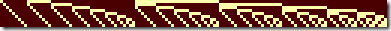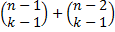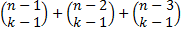## Friday, April 23, 2010

### Indexing and enumerating subsets of a given sizeI received an email yesterday from a gentleman named Calvin Miracle, asking my opinion on subset enumeration strategy. He also provided a copy of this paper, which details an algorithm to generate a sequence of subsets in Banker’s order1, and asked about an algorithm to generate these subsets in a stateless manner. I’ll let him describe it:

Given a call to the method banker(n,k,i), where n is the size of a set, k is the subset size under consideration, and i is the i'th subset of size k, the method will return a boolean vector of size n, with only k TRUE values, that selects the i'th k-size subset from the overall set.

Now, prior to this I had never heard of Banker’s sequences nor really thought about enumerating subsets, but I’m always willing to be nerd sniped so I gave it a go. Presented here is the algorithm I designed for him.

## Disclaimer

After writing this algorithm, I did some more digging and found out that some recent research has been done into enumerating subsets of a specific size, although in lexicographic order rather than Banker’s order. Wikipedia has details on this, and provides an algorithm very similar to the one I created here for except lexicographic ordering. So none of this should be seen as especially new or groundbreaking, although it was new to me and hopefully will be to you as well.

## Algorithm

The basic idea of this algorithm is that given any choice of the first element for our subset, we can calculate how many possible ways to choose the remaining elements there are, and we know that every subset with this first element will come before every subset with a later first element according to our ordering scheme. So we can iterate through the possible choices of first element, totalling up how many subsets each represents, until we find the range that the desired index falls within. We can then recursively determine the rest of the subset in the same manner.

So, Let n be the number of items, k the size of the subsequence, and i the specific index we are looking for. We will enumerate sequences by considering all subsets that start with “1”, then all that start with “01”, then all that start with “001”, etc., where “0” represents skipping an item and “1” represents selecting it. There are n-1 choose k-1 of the first sort, n-2 choose k-1 of the second, n-3 choose k-1 of the third, etc. So:

 “1”: 0 <= i <“01”:<= i <“001”:<= i <…

Once we know what prefix i has, we can recursively determine the next sequence using n' = n-(prefix length), k' = k-1, i' = i-(bottom of range).

## Example

Let n = 5, k = 3, i = 7:

 “1” 0 <= i < 6                        (4C2) “01”: 6 <= i < 9 = 6+3          (4C2 + 3C2)

So, initial prefix is “01”.

We recurse with n = 3, k = 2, i = 1:

 “1” 0 <= i < 2                        (2C1)

So, the next piece is “1”.

Finally, we recurse with n=2, k=1, i=1:
Trivially we can see that for “10” = 0, “01” = 1, so the last piece is “01”.

Putting this together, the sequence is “01101”,  so items 2, 3, and 5 of the 5 compose the 7th subset2 of length 3.

## Complexity

This algorithm is quite efficient – it needs to check at most n prefixes in total over all the levels of recursion, since each we consider shortens our candidate set by 1. There will be at most k recursive levels, and kn. So if we handwaive the “a choose b” calculations, we find that this can be done in O(n) time, which is O(1) in the size of the output, and thus optimal.

If we don’t handwaive the choice functions, we find that nCk is O(n!) in the worst case, so the result requires O(log(n!)) =O(nlogn) bits to store. If addition is O(1) we still may need to add up to n of these, so the total worst-case runtime is O(n2 logn), or O(nlogn) in the size of the output. However, with small values for k we don't get anywhere close to that worst case, and indeed are closer to O(logn) in size of the input. This is still quite efficient, and probably about the best that can be achieved in a function of this type. We should also consider the time needed to calculate the choice values, but if we are iterating over these indices the values can be pre-cached first and then the amortized cost per subsequence lookup goes to effectively zero.

## Code

If you want to play with this algorithm yourself, I have placed some java code implementing it on GitHub. It is as efficient as expected, and should be plenty fast enough for anything you could conceivably need it for. For example, it takes essentially no time to determine that the 160,000,000,000,000,000,000,000,000,000th subset of size 12 from a 10,000 item set is {0, 1, 2, 69, 1212, 1381, 4878, 5291, 5974, 6139, 6639, 8979}. So have at it!

Update: Ligon Liu has kindly provided a C# port of this code, which I have added to the repository (it the c# directory).

Update 2: Josiah Carlson has kindly provided a python port of this code, also in the repository now.

Update 3: Richard Lyon has kindly provided ports to both JavaScript and PHP, on GitHub as well. Thanks guys!

Update 4: Corin Lawson has a C implementation in his GitHub repository, along with other Banker's order functions. Great!

## Footnotes

1. The easiest way to think of Banker’s ordering is to think of comparing the indices of the items that make up the subsets. So to order two subsets, take the list of indices of elements in the first subset, and compare it to that of the second subset in standard dictionary (lexicographic) order. So {2, 3, 4} comes before {2, 3, 5} but after {1, 3, 5}, for example.

The image at the top of this post depicts the Banker’s ordering for subsets of  lengths 1, 2, and 3, respectively, from a set of size 9.
2. Subsets are indexed from 0, so you may consider this the 8th subset.

1.What really attracted me to Banker's was the way it enumerated subsets *monotonically*. My application was to find a maximal clique in an undirected graph. In a graph of 100 nodes (say), Banker's allowed me to eliminate all sets of size 100, then all sets of size 99, then all of 98, and so on. Banker's made *steady progress* to find a maximal clique, which no other count would do.

As I related to Eric, I was just surprised not to find Banker's as a standard recipe in all of the algorithm cookbooks that are out there. I credit Eric with being the smartest guy in the room for figuring out a static method for Banker's, and I hope Banker's becomes much better known and used.

-- Calvin Miracle, Louisville KY

2.> in a stateless manor
Probably, "in a stateless manner"

3.Good catch, thanks.

4.Interesting article, thanks! I plan to use this technique in the future, to iterate over subsets. I played around with writing some Haskell code to implement this an I blogged about it at http://alexstangl.wordpress.com/2010/04/27/finding-minimum-qualified-subset-in-haskell/

I just reviewed the comments and noticed Calvin's application is to find a maximal, not minimal subset. I'll add that capability into the Haskell code.

5.Hacked together a version in Python, it's yours if you want it.

6.That'd be great, sure. You can send it to me however you like, and I'll add it to the repository with the other languages. Thanks!

7.Hi Alex,

I figure that a *maximal subset* that IS selected is the same as a *minimal subset* that is NOT selected.. In other words, invert the bits in the boolean vector.. This reflects the symmetry of the C(n, k) function, so your function can count either UP or DOWN..

-- Cal in Louisville

8.How many ways can one choose a k-tuple of disjoint subsets of an n element set A?

9.Thanks again Richard, the PHP code and the JavaScript version you sent me are both up on GitHub now :).

10.Great article! Here's my implementation in C (sorry, no big int):
unsigned int compute (unsigned int n, unsigned int k, unsigned int i){    unsigned int binom, b = 0;    do {        if (i == 0 || (binom = choose(n - 1, k - 1)) > i)            k--, b |= 1;        else            i -= binom;        b <<= 1;    } while (--n && k && ((b <<= 1) || 1));    b <<= n;    return b;}

Complete code here: https://github.com/au-phiware/bankers
I also have an implementation that doesn't use nCk (but it's not as efficient).

1.Sorry for the long delay Corin, and thanks for the code! I've added it to the post :).

11.Thanks very much for this - I was running into performance issues with trying to generate large Kneser's graphs due to the requirement for enumerating subsets - this has sped up it up massively.

I ported it to PHP and added an "all" method - seems to be running very fast! It's listed at http://richardlyon.co.uk/enum.php - feel free to add it to the github repo if you want!